Con­sider the Schrö­din­ger equa­tion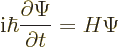If the Hamil­ton­ian is in­de­pen­dent of time, the so­lu­tion can be writ­ten in terms of the Hamil­ton­ian en­ergy eigen­val­ues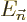and eigen­func­tions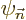as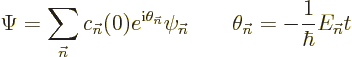Here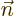stands for the quan­tum num­bers of the eigen­func­tions and the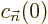are ar­bi­trary con­stants.

How­ever, the Hamil­ton­ian varies with time for the sys­tems of in­ter­est here. Still, at any given time its eigen­func­tions form a com­plete set. So it is still pos­si­ble to write the wave func­tion as a sum of them, say like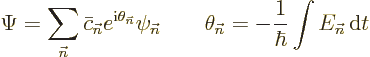(D.18)

But the co­ef­fi­cients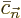can no longer be as­sumed to be con­stant like the. They may be dif­fer­ent at dif­fer­ent times.

To get an equa­tion for their vari­a­tion, plug the ex­pres­sion for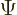in the Schrö­din­ger equa­tion. That gives: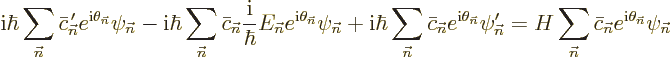where the primes in­di­cate time de­riv­a­tives. The mid­dle sum in the left hand side and the right hand side can­cel against each other since by de­f­i­n­i­tionis an eigen­func­tion of the Hamil­ton­ian with eigen­value. For the re­main­ing two sums, take an in­ner prod­uct with an ar­bi­trary eigen­func­tion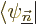: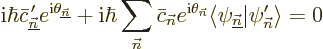In the first sum only the term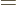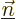sur­vived be­cause of the or­tho­nor­mal­ity of the eigen­func­tions. Di­vide by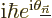and re­arrange to get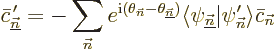(D.19)

This is still ex­act.

How­ever, the pur­pose of the cur­rent de­riva­tion is to ad­dress the adi­a­batic ap­prox­i­ma­tion. The adi­a­batic ap­prox­i­ma­tion as­sumes that the en­tire evo­lu­tion takes place very slowly over a large time in­ter­val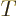. For such an evo­lu­tion, it helps to con­sider all quan­ti­ties to be func­tions of the scaled time vari­able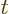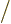. Vari­ables change by a fi­nite amount whenchanges by a fi­nite frac­tion of, so whenchanges by a fi­nite amount. This im­plies that the time de­riv­a­tives of the slowly vary­ing quan­ti­ties are nor­mally small, of or­der 1/.

Con­sider now first the case that there is no de­gen­er­acy, in other words, that there is only one eigen­func­tionfor each en­ergy. If the Hamil­ton­ian changes slowly and reg­u­larly in time, then so do the en­ergy eigen­val­ues and eigen­func­tions. In par­tic­u­lar, the time de­riv­a­tives of the eigen­func­tions in (D.19) are small of or­der 1/. It then fol­lows from the en­tire equa­tion that the time de­riv­a­tives of the co­ef­fi­cients are small of or­der 1too.

(Re­call that the square mag­ni­tudes of the co­ef­fi­cients give the prob­a­bil­ity for the cor­re­spond­ing en­ergy. So the mag­ni­tude of the co­ef­fi­cients is bounded by 1. Also, for sim­plic­ity it will be as­sumed that the num­ber of eigen­func­tions in the sys­tem is fi­nite. Oth­er­wise the sums overmight ex­plode. This book rou­tinely as­sumes that it is good enough to ap­prox­i­mate an in­fi­nite sys­tem by a large-enough fi­nite one. That makes life a lot eas­ier, not just here but also in other de­riva­tions like {D.18}.)

It is con­ve­nient to split up the sum in (D.19):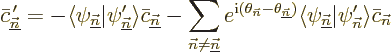(D.20)

Un­der the stated con­di­tions, the fi­nal sum can be ig­nored.

How­ever, that is not be­cause it is small due to the time de­riv­a­tive in it, as one ref­er­ence claims. While the time de­riv­a­tive ofis in­deed small of or­der 1/, it acts over a time that is large of or­der. The sum can be ig­nored be­cause of the ex­po­nen­tial in it. As the de­f­i­n­i­tion of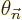shows, it varies on the nor­mal time scale, rather than on the long time scale. There­fore it os­cil­lates many times on the long time scale; that causes op­po­site val­ues of the ex­po­nen­tial to largely can­cel each other.

To show that more pre­cisely, note that the for­mal so­lu­tion of the full equa­tion (D.20) is, [41, 19.2]: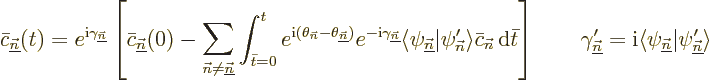(D.21)

To check this so­lu­tion, you can just plug it in. Note in do­ing so that the in­te­grands are taken to be func­tions of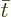, not.

All the in­te­grals are neg­li­gi­bly small be­cause of the rapid vari­a­tion of the first ex­po­nen­tial in them. To ver­ify that, rewrite them a bit and then per­form an in­te­gra­tion by parts: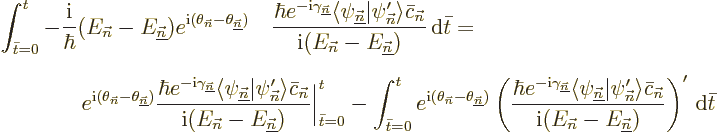The first term in the right hand side is small of or­der 1be­cause the time de­riv­a­tive of the wave func­tion is. The in­te­grand in the sec­ond term is small of or­der 1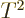be­cause of the two time de­riv­a­tives. So in­te­grated over an or­dertime range, it is small of or­der 1like the first term. It fol­lows that the in­te­grals in (D.21) be­come zero in the limit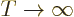.

And that means that in the adi­a­batic ap­prox­i­ma­tion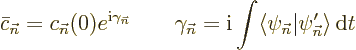The un­der­bar used to keepandapart is no longer needed here since only one set of quan­tum num­bers ap­pears. This ex­pres­sion for the co­ef­fi­cients can be plugged in (D.18) to find the wave func­tion. The con­stantsde­pend on the ini­tial con­di­tion for. (They also de­pend on the choice of in­te­gra­tion con­stants forand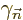, but nor­mally you take the phases zero at the ini­tial time).

Note thatis real. To ver­ify that, dif­fer­en­ti­ate the nor­mal­iza­tion re­quire­ment to get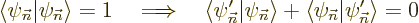So the sum of the in­ner prod­uct plus its com­plex con­ju­gate are zero. That makes it purely imag­i­nary, sois real.

Since bothandare real, it fol­lows that the mag­ni­tudes of the co­ef­fi­cients of the eigen­func­tions do not change in time. In par­tic­u­lar, if the sys­tem starts out in a sin­gle eigen­func­tion, then it stays in that eigen­func­tion.

So far it has been as­sumed that there is no de­gen­er­acy, at least not for the con­sid­ered state. How­ever it is no prob­lem if at a fi­nite num­ber of times, the en­ergy of the con­sid­ered state crosses some other en­ergy. For ex­am­ple, con­sider a three-di­men­sion­al har­monic os­cil­la­tor with three time vary­ing spring stiff­nesses. When­ever any two stiff­nesses be­come equal, there is sig­nif­i­cant de­gen­er­acy. De­spite that, the given adi­a­batic so­lu­tion still ap­plies. (This does as­sume that you have cho­sen the eigen­func­tions to change smoothly through de­gen­er­acy, as per­tur­ba­tion the­ory says you can, {D.79}.)

To ver­ify that the so­lu­tion is in­deed still valid, cut out a time in­ter­val of size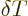around each cross­ing time. Here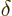is some num­ber still to be cho­sen. The parts of the in­te­grals in (D.21) out­side of these in­ter­vals have mag­ni­tudes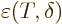that be­come zero whenfor the same rea­sons as be­fore. The parts of the in­te­grals cor­re­spond­ing to the in­ter­vals can be es­ti­mated as no more than some fi­nite mul­ti­ple of. The rea­son is that the in­te­grands are of or­der 1and they are in­te­grated over ranges of size. All to­gether, that is enough to show that the com­plete in­te­grals are less than say 1%; just takesmall enough that the in­ter­vals con­tribute no more than 0.5% and then takelarge enough that the re­main­ing in­te­gra­tion range con­tributes no more than 0.5% too. Since you can play the same game for 0.1%, 0.01% or any ar­bi­trar­ily small amount, the con­clu­sion is that for in­fi­nite, the con­tri­bu­tion of the in­te­grals be­comes zero. So in the limit, the adi­a­batic so­lu­tion ap­plies.

Things change if some en­ergy lev­els are per­ma­nently de­gen­er­ate. Con­sider an har­monic os­cil­la­tor for which at least two spring stiff­nesses are per­ma­nently equal. In that case, you need to solve for all co­ef­fi­cients at a given en­ergy level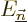to­gether. To fig­ure out how to do that, you will need to con­sult a book on math­e­mat­ics that cov­ers sys­tems of or­di­nary dif­fer­en­tial equa­tions. In par­tic­u­lar, the co­ef­fi­cient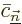in (D.21) gets re­placed by a vec­tor of co­ef­fi­cients with the same en­ergy. The scalar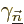be­comes a ma­trix with in­dices rang­ing over the set of co­ef­fi­cients in the vec­tor. Also,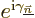gets re­placed by a fun­da­men­tal so­lu­tion ma­trix, a ma­trix con­sist­ing of in­de­pen­dent so­lu­tion vec­tors. And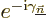is the in­verse ma­trix. The sum no longer in­cludes any of the co­ef­fi­cients of the con­sid­ered en­ergy.

More re­cent de­riva­tions al­low the spec­trum to be con­tin­u­ous, in which case the nonzero en­ergy gaps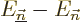can no longer be as­sumed to be larger than some nonzero amount. And un­for­tu­nately, as­sum­ing the sys­tem to be ap­prox­i­mated by a fi­nite one helps only par­tially here; an ac­cu­rate ap­prox­i­ma­tion will pro­duce very closely spaced en­er­gies. Such prob­lems are well out­side the scope of this book.